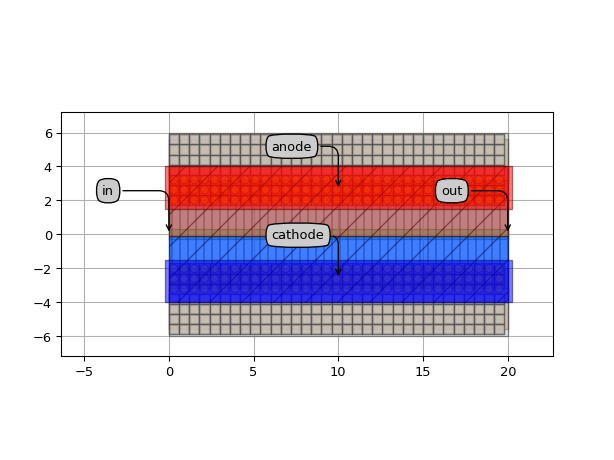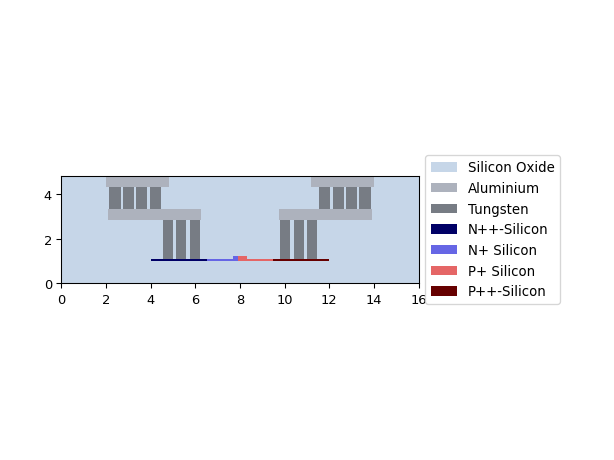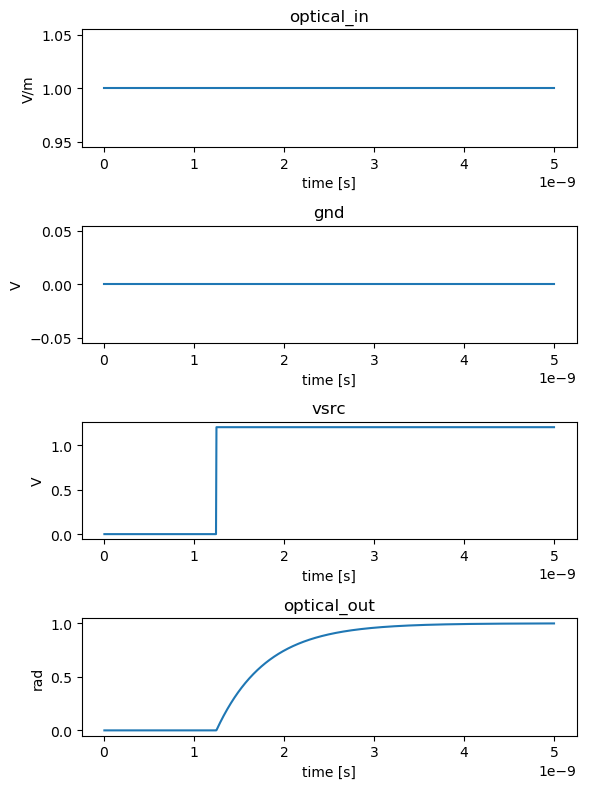# Phase shifters¶

SiFab contains electro-optic components that modulate the phase of the light when a voltage difference is applied:

The section Model and simulation recipes explains how to simulate the phase shifter.

## PhaseShifterWaveguide¶

This PCell is an electro-optical waveguide with adjustable length. The p-n junction is centered in the middle of the optical waveguide and can be offset by tuning the property junction_offset. Many other properties can be controlled and are listed in the PCell reference.

Reference

Click on the name of the component below to see the complete PCell reference.

 si_fab.all.PhaseShifterWaveguide Phase shifter waveguide with a lateral p-n junction.

Example

from si_fab import all as pdk
from ipkiss3 import all as i3

# Phase Shifter
ps = pdk.PhaseShifterWaveguide(
length=20.0,
core_width=0.6,
rib_width=7.8,
junction_offset=-0.1,
p_width=4.1,
pplus_offset=1.5,
pplus_extension=0.25,
n_width=3.9,
nplus_offset=1.5,
nplus_extension=0.25,
p_contact_rows=3,
n_contact_rows=3,
p_contact_offset=2.025,
n_contact_offset=2.025,
contact_pitch=0.6,
m1_width=3.6,
m1_offset=2.0,
m2_width=2.8,
m2_offset=3.2,
n_via_rows=4,
via_pitch=0.6,
via_offset=3.8,
)

ps_lv = ps.Layout()
ps_lv.visualize(annotate=True)
xs = ps_lv.cross_section(cross_section_path=i3.Shape([(1.0, -8.0), (1.0, 8.0)]))
xs.visualize()
ps_lv.write_gdsii("ps.gds")## Model and simulation recipes¶

The phase of the waveguide is calculated using the effective index ($$n_{eff}$$) of the trace template (trace_template) used to draw the waveguide. This is a Rib waveguide templates template, and is controlled with the core_width and rib_width properties of the PhaseShifterWaveguide.

The model of the heater adds a phase modulation to the phase of the waveguide that is calculated as follows:

$\Delta \Phi = \frac{\pi L V_d}{ V_{\pi} L_{\pi}}$

where

• $$L$$ is the length of the phase shifter;
• $$V_d$$ is the voltage drop over the phase shifter junction;
• $$V_{\pi} L_{\pi}$$ is the efficiency of the phase shifter.

The voltage $$V_d$$ is associated with a delay $$\tau$$ that is, in first approximation, equal to $$RC$$. The resistance $$R$$ equals the output impedance of the driving electronics. The capacitance $$C$$ equals the total electrical capacitance over the junction, which is usually dominated by the junction capacitance. This model does not account for the voltage dependence of $$V_{\pi} L_{\pi}$$ and $$\tau$$. In addition, their values are not linked to the layout and need to be calculated or measured independently.

Using the simulation recipe simulate_phaseshifter, one can easily check the phase shift achieved by a specified voltage, as well as the speed to achieve this response. Here we apply a voltage of $$V_{\pi} L_{\pi}$$ V on a modulator that has length of 1 cm, which gives a phase shift of $$\pi$$.

Reference

Click on the name of the function below to see the complete API reference of the simulation recipe.

 si_fab.components.phase_shifter.simulation.simulate.simulate_phaseshifter Simulation recipe to simulate the phase shifter.

Example

from si_fab import all as pdk
from si_fab.components.phase_shifter.simulation.simulate import simulate_phaseshifter
import pylab as plt
import numpy as np
import os

# Phase Shifter
name = "simple_step"
results_array = []
length = 1e4
ps = pdk.PhaseShifterWaveguide(length=length,
core_width=0.5,
rib_width=7.8,
junction_offset=-0.1)
vpi_lpi = 1.2  # V.cm
capacitance = 1.1e-15  # F/um
resistance = 50  # Ohm
tau = ps.length * capacitance * resistance
ps.CircuitModel(vpi_lpi=vpi_lpi)

results = simulate_phaseshifter(
cell=ps,
nsteps=1000,
dt=5e-12,
step_amplitude=vpi_lpi,
center_wavelength=1.5,
debug=False,
)

def phase_unwrap_normalize(results):
unwrapped = np.unwrap(np.angle(results))
return (unwrapped - unwrapped * np.ones(unwrapped.shape)) / np.pi

outputs = ["optical_in", "gnd", "vsrc_mod", "optical_out"]
titles = ["Source input", "Source ground", "Source modulation", "Output"]
process = [np.real, np.real, np.real, phase_unwrap_normalize]
ylabels = ["V/m", "V", "V", "rad"]
fig, axs = plt.subplots(nrows=len(outputs), ncols=1, figsize=(6, 8))

times = results.timesteps[1:]

for axes, fproc, output, ylabel in zip(axs, process, outputs, ylabels):
signal = fproc(results[output])[1:]
axes.set_title(output)
axes.plot(times, signal)
axes.set_ylabel(ylabel)
axes.set_xlabel("time [s]")

plt.tight_layout()
fig.savefig(os.path.join("{}.png".format(name)), bbox_inches='tight')
plt.show()